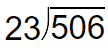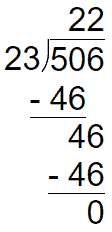# 3-digit by 2-digit Division Worksheets

## All About These 15 Worksheets

These 3-digit by 2-digit Division worksheets are designed to help students master the concept of dividing three-digit numbers by two-digit numbers. The worksheets include a variety of creative and engaging exercises to help students build their skills and confidence in division.

Each worksheet in the series includes a mix of problems with varying degrees of difficulty, designed to challenge and engage students at different skill levels. The problems require students to divide three-digit numbers by two-digit numbers with and without remainders.

These worksheets also provide ample space for students to show their work and write down their answers. This helps them develop their problem-solving skills and allows for feedback from teachers or peers.

This series is most suitable for students in grades 5 to 7. It can be used in the classroom as a supplement to the math curriculum or at home as a way for parents to help their children practice and reinforce their math skills.

Overall, these 3-digit by 2-digit Division worksheets provide a comprehensive and engaging way for students to develop their skills and confidence in dividing three-digit numbers by two-digit numbers. With these worksheets, students can build a solid foundation in math and develop the skills they need to succeed in higher-level math courses.

## How to Solve These Types of Problems

Dividing a 3-digit number by a 2-digit number is a multi-step process. Here’s a step-by-step guide that teachers can share with their students.

Step 1: Write Down the Problem

The dividend goes inside the bracket. The divisor is aligned to the left, just outside the bracket.Step 2: How Many Times?

Determine how many times the divisor (23) goes into the first digit(s) of the dividend (506). In this case, 23 does not go into 5, so we move to the next digit and consider the first two digits: 50.

23 goes into 50 two times. Write down the “2” above the second digit of the dividend (in this case, above 0):

Step 3: Setup and Process Remaining Dividend

After Step 2, we are left with a remainder of 4. We drop that remainder down and drop the remaining portion of the quotient. This results in a remaining dividend of 46.  We therefore divide 46 by 23 = 2.The results in a final quotient of 22.